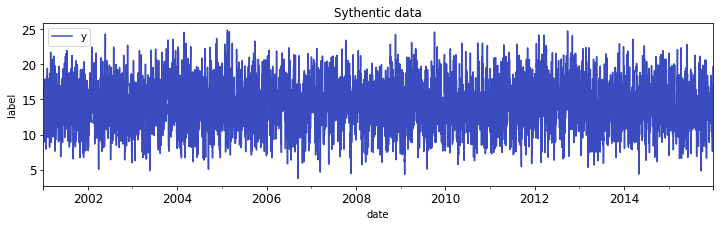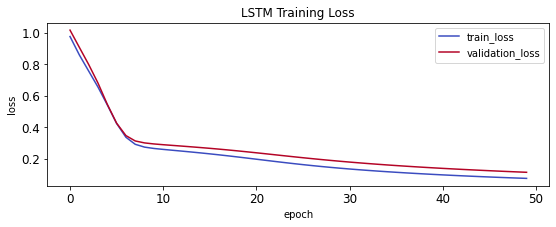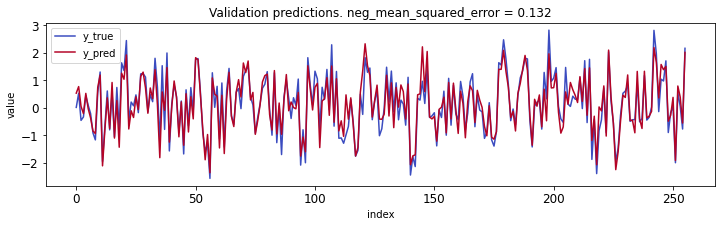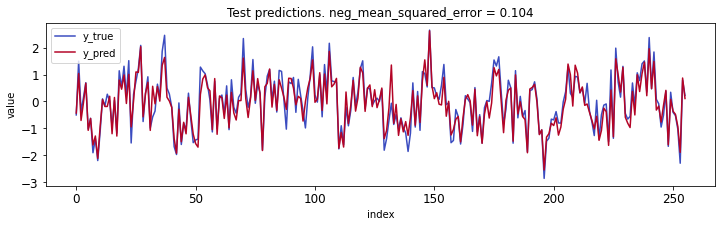# LSTM forecasting with Synthetic Data¶

Credits

## Dependencies¶

In :
import datetime
import numpy as np
import os
import sys
import tensorflow as tf
from tensorflow import keras
print(tf.__version__)
print(sys.version)

2.7.0
3.7.12 (default, Sep 10 2021, 00:21:48)
[GCC 7.5.0]


### Version specific dependencies¶

Requires run time restart

In :
!pip install pandas==1.1.5 # requires Runtime restart
!pip install scikit-learn==1.0 # requires Runtime restart
import pandas as pd
assert pd.__version__ == "1.1.5"
pd.set_option("max_columns", 100)
import sklearn
assert sklearn.__version__ == "1.0"
from sklearn import metrics
from sklearn.base import BaseEstimator, TransformerMixin
from sklearn.datasets import make_friedman1, make_regression
from sklearn.impute import SimpleImputer
from sklearn.pipeline import FeatureUnion, Pipeline
from sklearn.preprocessing import StandardScaler, OneHotEncoder
from sklearn.model_selection import GridSearchCV, TimeSeriesSplit

Requirement already satisfied: pandas==1.1.5 in /usr/local/lib/python3.7/dist-packages (1.1.5)
Requirement already satisfied: numpy>=1.15.4 in /usr/local/lib/python3.7/dist-packages (from pandas==1.1.5) (1.19.5)
Requirement already satisfied: pytz>=2017.2 in /usr/local/lib/python3.7/dist-packages (from pandas==1.1.5) (2018.9)
Requirement already satisfied: python-dateutil>=2.7.3 in /usr/local/lib/python3.7/dist-packages (from pandas==1.1.5) (2.8.2)
Requirement already satisfied: six>=1.5 in /usr/local/lib/python3.7/dist-packages (from python-dateutil>=2.7.3->pandas==1.1.5) (1.15.0)
Requirement already satisfied: scikit-learn==1.0 in /usr/local/lib/python3.7/dist-packages (1.0)
Requirement already satisfied: joblib>=0.11 in /usr/local/lib/python3.7/dist-packages (from scikit-learn==1.0) (1.1.0)
Requirement already satisfied: numpy>=1.14.6 in /usr/local/lib/python3.7/dist-packages (from scikit-learn==1.0) (1.19.5)
Requirement already satisfied: scipy>=1.1.0 in /usr/local/lib/python3.7/dist-packages (from scikit-learn==1.0) (1.4.1)

In :
np.random.seed(1)


In :
forecast_steps = 7 # 7

In :
def generate_data():
df = pd.DataFrame()
start_date = datetime.datetime(year=2001, month=1, day=1)
df["date"] = [start_date + datetime.timedelta(days=x+1) for x in range(365*15)]
num_features = 10 # only 5 features determine label
n_informative = 5 # determine the label, the rest is random
X, y = make_friedman1(n_samples=df.shape, n_features=num_features, noise=1.0)
# X, y = make_regression(n_samples=df.shape, n_features=num_features, n_informative=n_informative, n_targets=1)
df.loc[:, [f"x_{i}" for i in range(num_features)]] = X
df.loc[:, "x_2"] *= 20 # scale feature
df.loc[:, "x_3"] *= 30 # scale feature
df.loc[:, "x_7"] *= 70 # scale feature
df.loc[:, "x_8"] *= 80 # scale feature
df.loc[:, "y"] = y
df.loc[:, "y"] = (
0.5 * df.loc[:, "y"] +
0.5 * df.loc[:, "y"].rolling(window=3).mean()
)
df.loc[:, "y"] = df.loc[:, "y"].shift(forecast_steps)
df.rolling(2, min_periods=1).sum()
return df
df = generate_data()
df.describe(include="all", datetime_is_numeric=True)

Out:
date x_0 x_1 x_2 x_3 x_4 x_5 x_6 x_7 x_8 x_9 y
count 5475 5475.000000 5475.000000 5475.000000 5475.000000 5475.000000 5475.000000 5475.000000 5475.000000 5475.000000 5475.000000 5466.000000
mean 2008-07-01 00:00:00.000029184 0.499200 0.500893 9.947641 14.926298 0.502209 0.501965 0.491453 35.024637 39.932751 0.506453 14.366396
min 2001-01-02 00:00:00 0.000084 0.000079 0.002287 0.001414 0.000072 0.000248 0.000079 0.017472 0.000830 0.000011 3.783890
25% 2004-10-01 12:00:00 0.251183 0.244304 4.976404 7.273625 0.252892 0.248793 0.239705 17.336051 20.410696 0.249481 11.948152
50% 2008-07-01 00:00:00 0.491677 0.504741 9.950743 14.968018 0.506688 0.500906 0.492554 35.429179 39.796218 0.519164 14.439272
75% 2012-03-30 12:00:00 0.747067 0.758768 14.887439 22.626872 0.751767 0.759172 0.737345 52.544612 59.656927 0.755871 16.754376
max 2015-12-29 00:00:00 0.999754 0.999834 19.995147 29.995535 0.999900 0.999990 0.999660 69.967756 79.957545 0.999813 24.853195
std NaN 0.285983 0.291814 5.760796 8.751435 0.288316 0.289881 0.287323 20.240193 22.963125 0.289597 3.484810
In :
df.head(10)

Out:
date x_0 x_1 x_2 x_3 x_4 x_5 x_6 x_7 x_8 x_9 y
0 2001-01-02 0.417022 0.720324 0.002287 9.069977 0.146756 0.092339 0.186260 24.189251 31.741398 0.538817 NaN
1 2001-01-03 0.419195 0.685220 4.089045 26.343523 0.027388 0.670468 0.417305 39.108288 11.230955 0.198101 NaN
2 2001-01-04 0.800745 0.968262 6.268484 20.769678 0.876389 0.894607 0.085044 2.733835 13.586434 0.878143 NaN
3 2001-01-05 0.098347 0.421108 19.157791 15.994959 0.691877 0.315516 0.686501 58.423797 1.463062 0.750144 NaN
4 2001-01-06 0.988861 0.748166 5.608880 23.678380 0.103226 0.447894 0.908596 20.552990 23.022027 0.130029 NaN
5 2001-01-07 0.019367 0.678836 4.232562 7.966400 0.491573 0.053363 0.574118 10.271000 47.144443 0.699758 NaN
6 2001-01-08 0.102334 0.414056 13.888003 12.425378 0.049953 0.535896 0.663795 36.042238 75.567580 0.586555 NaN
7 2001-01-09 0.903402 0.137475 2.785527 24.221739 0.397677 0.165354 0.927509 24.343610 60.064968 0.725998 NaN
8 2001-01-10 0.883306 0.623672 15.018849 10.466950 0.269928 0.895886 0.428091 67.538803 53.075320 0.621696 NaN
9 2001-01-11 0.114746 0.949489 8.998243 17.351688 0.408137 0.237027 0.903380 40.157564 0.229626 0.617145 16.564334
In :
df.plot(kind="line",
x="date",
y="y",
colormap="coolwarm",
figsize=(12, 3),
fontsize=12,
title="Sythentic data",
xlabel="date",
ylabel="label")

Out:
<matplotlib.axes._subplots.AxesSubplot at 0x7f869d0251d0>In :
df_corr = df.copy(deep=True)
df_corr.loc[:, "future_label"] = df_corr.loc[:, "y"].shift(-forecast_steps)

Out:
x_0 x_1 x_2 x_3 x_4 x_5 x_6 x_7 x_8 x_9 y future_label
x_0 1.000000 -0.011230 -0.008236 -0.003817 0.008050 -0.007087 -0.005471 -0.018666 0.002469 -0.007974 0.013433 0.343410
x_1 -0.011230 1.000000 0.013938 -0.022951 0.007660 0.001471 -0.005772 0.007867 0.000011 -0.001630 -0.000411 0.367546
x_2 -0.008236 0.013938 1.000000 -0.005004 -0.010491 -0.003633 0.017680 -0.018911 -0.017886 0.010443 -0.012752 -0.004859
x_3 -0.003817 -0.022951 -0.005004 1.000000 -0.009876 0.036063 -0.010286 -0.002176 -0.009020 0.007870 -0.007557 0.543532
x_4 0.008050 0.007660 -0.010491 -0.009876 1.000000 0.007150 0.027339 -0.005743 0.009159 0.006346 0.011170 0.280323
x_5 -0.007087 0.001471 -0.003633 0.036063 0.007150 1.000000 -0.017550 0.018368 -0.009455 0.010404 0.026603 0.013868
x_6 -0.005471 -0.005772 0.017680 -0.010286 0.027339 -0.017550 1.000000 0.001568 -0.018141 -0.017902 0.007078 -0.003980
x_7 -0.018666 0.007867 -0.018911 -0.002176 -0.005743 0.018368 0.001568 1.000000 -0.006124 -0.016254 -0.014022 -0.006538
x_8 0.002469 0.000011 -0.017886 -0.009020 0.009159 -0.009455 -0.018141 -0.006124 1.000000 -0.019908 -0.005104 0.009448
x_9 -0.007974 -0.001630 0.010443 0.007870 0.006346 0.010404 -0.017902 -0.016254 -0.019908 1.000000 0.012354 -0.002757
y 0.013433 -0.000411 -0.012752 -0.007557 0.011170 0.026603 0.007078 -0.014022 -0.005104 0.012354 1.000000 -0.008424
future_label 0.343410 0.367546 -0.004859 0.543532 0.280323 0.013868 -0.003980 -0.006538 0.009448 -0.002757 -0.008424 1.000000

## Train / validation / test split¶

Lookback & forecast step example:

• lookback_steps = 12
• forecast_steps = 6
• features with row indexes 0:11 will be used to forecast label from row 17
In :
lookback_steps = 14 # length of history provided to learn label at t
end_train_idx = int(0.7 * df.shape)
end_validation_idx = int(0.85 * df.shape)
print(f"""train idx: [{df.index}, {end_train_idx})
validation idx: [{end_train_idx}, {end_validation_idx})
test idx: [{end_validation_idx}-{df.shape})""")

train idx: [0, 3832)
validation idx: [3832, 4653)
test idx: [4653-5475)


## Transform¶

Using a pipeline to ensure no train/test data leakage occurs. Accomplished by:

• train set being transformed using fit_transform
• validation & test sets are being transformed using transform only
In :
# label 'y' is included as a feature because historical labels are provided as features
numerical_features = ["y"] + [f"x_{i}" for i in range(10)]

In :
def get_feature_pipeline():
class FeatureSelector(BaseEstimator, TransformerMixin):
def __init__(self, feature_names):
self.feature_names = feature_names
def fit( self, X, y = None ):
return self
def transform(self, X, y=None):
return X.loc[:, self.feature_names].copy(deep=True)
numerical_pipeline = Pipeline(steps = [
("num_selector", FeatureSelector(numerical_features)),
("imputer", SimpleImputer(strategy="median")),
("std_scaler", StandardScaler())
])
feature_pipeline = FeatureUnion(
n_jobs=1,
transformer_list=[
("numerical_pipeline", numerical_pipeline),
# ("categorical_pipeline", categorical_pipeline),
]
)
return feature_pipeline


### Train¶

In :
feature_pipeline = get_feature_pipeline()
train_df = pd.DataFrame(
feature_pipeline.fit_transform(
df.loc[:end_train_idx, :]
),
columns=numerical_features
)
# Train label is offset by the number of steps into the future we're forecasting
train_df.loc[:, "label"] = train_df.loc[:, "y"].shift(-forecast_steps)
train_df = train_df.loc[train_df["label"].notna(), :]
assert 0 == train_df.isna().sum().sum()
print(train_df.shape)

(3826, 12)

Out:
y x_0 x_1 x_2 x_3 x_4 x_5 x_6 x_7 x_8 x_9 label
0 0.019987 -0.281535 0.743078 -1.732444 -0.670609 -1.240892 -1.392544 -1.048232 -0.518341 -0.363001 0.105768 0.019987
1 0.019987 -0.273918 0.623383 -1.021729 1.306435 -1.656933 0.605012 -0.244852 0.215292 -1.254779 -1.065334 0.019987
2 0.019987 1.063775 1.588445 -0.642710 0.668480 1.302139 1.379459 -1.400177 -1.573396 -1.152365 1.272093 0.638336
3 0.019987 -1.398792 -0.277134 1.598828 0.121990 0.659049 -0.621421 0.691189 1.165119 -1.679479 0.832140 0.263882
4 0.019987 1.723301 0.838005 -0.757420 1.001396 -1.392609 -0.164027 1.463449 -0.697151 -0.742112 -1.299313 1.021163
In :
dataset_train = keras.preprocessing.timeseries_dataset_from_array(
data=train_df.loc[:train_df.shape-lookback_steps, numerical_features],
targets=train_df.loc[lookback_steps-1:, "label"],
sequence_length=lookback_steps,
sequence_stride=1,
sampling_rate=1,
batch_size=256,
shuffle=False,
)
for x, y in dataset_train.take(1):
print(x.shape, y.shape)

(256, 14, 11) (256,)


### Validation¶

In :
def df_transform_to_keras_dataset(df_idx_start, df_idx_end):
validation_df = pd.DataFrame(
feature_pipeline.transform(
df.loc[df_idx_start:df_idx_end, :]
),
columns=numerical_features
)
validation_df.loc[:, "label"] = validation_df.loc[:, "y"].shift(-forecast_steps)
validation_df = validation_df.loc[validation_df["label"].notna(), :]
assert 0 == validation_df.isna().sum().sum()
print(validation_df.shape)
dataset_validation = keras.preprocessing.timeseries_dataset_from_array(
data=validation_df.loc[:validation_df.shape-lookback_steps, numerical_features],
targets=validation_df.loc[lookback_steps-1:, "label"],
sequence_length=lookback_steps,
batch_size=256,
shuffle=False,
)
return dataset_validation
dataset_validation = df_transform_to_keras_dataset(end_train_idx, end_validation_idx)
for x, y in dataset_validation.take(1):
print(x.shape, y.shape)

(815, 12)
(256, 14, 11) (256,)


### Verify transforms¶

In :
print(f"target label: {y}")
for i in range(len(x)):
print(f"y{i}: {x[i]}, x_0: {x[i]}, x_1: {x[i]}, x_-1: {x[i][-1]}")

target label: 0.019389618274903233
y0: 0.3897786614966334, x_0: -1.1019673030768893, x_1: -0.22405404433829246, x_-1: -0.9570927677004241
y1: 0.16253865446542173, x_0: 1.2114164259589433, x_1: -1.3445111874070004, x_-1: 1.5001103110847434
y2: -1.4409742610336096, x_0: 0.9761430256738434, x_1: 0.4193617145263754, x_-1: 0.6141486952660598
y3: -0.755023105235806, x_0: -0.8440149032050653, x_1: -1.025479123155977, x_-1: 0.03353121581481265
y4: -0.2880803134443823, x_0: -1.6817742306143941, x_1: -1.4613436867621472, x_-1: -0.6023766153506032
y5: 0.15952488527276376, x_0: -1.2653908970021783, x_1: 1.0120946328845757, x_-1: 0.33908976903160976
y6: 0.4354598032438619, x_0: 0.12260467256003346, x_1: -0.24265114930045203, x_-1: -1.2539298986455685
y7: 0.5935497893926679, x_0: -1.0379963619208725, x_1: 0.7004641382053569, x_-1: 1.1098251989723393
y8: -0.3899682030593925, x_0: -0.6986635312594595, x_1: 0.7927568983351336, x_-1: 1.3245205052542632
y9: 1.2179114273241878, x_0: 0.5238443966524786, x_1: -0.4999483464680014, x_-1: 0.22896240421404235
y10: -0.08175111391879324, x_0: -1.712561742804729, x_1: -1.6360440890929577, x_-1: 0.6876443875590319
y11: -2.0715253248394574, x_0: -0.8647974467917974, x_1: 1.1455358285234243, x_-1: 1.0368044056699262
y12: -0.7749879487852037, x_0: 0.3971075977013075, x_1: -1.166809983434937, x_-1: -0.06087473037660358
y13: -0.2119881874089851, x_0: 0.9152801589653095, x_1: 0.7976423263300022, x_-1: 0.3662436780133264

In :
print(f"Row idx of first label (see 'Name: x') in column 0:")
(
pd.DataFrame(feature_pipeline.transform(
df.loc[end_train_idx:end_validation_idx, :])
).loc[lookback_steps + forecast_steps - 1, :]
)

Row idx of first label (see 'Name: x') in column 0:

Out:
0     0.019390
1    -0.093626
2    -1.636704
3     0.594119
4    -0.120487
5     1.354507
6    -0.900181
7    -0.208709
8    -0.787929
9     1.055315
10   -0.603038
Name: 20, dtype: float64
In :
print(f"Rows idx of dataset to learn first label: 0:{lookback_steps - 1}")
(
pd.DataFrame(feature_pipeline.transform(
df.loc[end_train_idx:end_validation_idx, :])
).loc[lookback_steps-5:lookback_steps-1,:]
)

Rows idx of dataset to learn first label: 0:13

Out:
0 1 2 3 4 5 6 7 8 9 10
9 1.217911 0.523844 -0.499948 -0.396952 -0.106933 -0.593192 0.354691 0.977050 -1.455930 -0.195214 0.228962
10 -0.081751 -1.712562 -1.636044 -0.017625 -1.693798 -0.878965 -0.088157 1.244197 1.245123 1.138779 0.687644
11 -2.071525 -0.864797 1.145536 0.840013 0.320359 -0.920446 0.952525 0.120276 0.796968 0.406951 1.036804
12 -0.774988 0.397108 -1.166810 -1.360880 -0.725304 1.160456 0.122417 1.302718 -0.629019 -1.664261 -0.060875
13 -0.211988 0.915280 0.797642 -1.626310 -0.880756 -0.952941 1.273095 0.707316 0.690538 -1.044318 0.366244

## Model¶

In :
inputs = keras.layers.Input(shape=(x.shape, x.shape))
lstm_out = keras.layers.LSTM(32)(inputs)
outputs = keras.layers.Dense(1)(lstm_out)
model = keras.Model(inputs=inputs, outputs=outputs)
learning_rate=0.001),
loss="mse"
)
model.summary()

Model: "model"
_________________________________________________________________
Layer (type)                Output Shape              Param #
=================================================================
input_1 (InputLayer)        [(None, 14, 11)]          0

lstm (LSTM)                 (None, 32)                5632

dense (Dense)               (None, 1)                 33

=================================================================
Total params: 5,665
Trainable params: 5,665
Non-trainable params: 0
_________________________________________________________________

In :
model_name = "lstm_synthetic_data"
path_checkpoint = f"/content/drive/My Drive/Colab Notebooks/forecasting/{model_name}.h5"
es_callback = keras.callbacks.EarlyStopping(monitor="val_loss", min_delta=0, patience=5)

modelckpt_callback = keras.callbacks.ModelCheckpoint(
monitor="val_loss",
filepath=path_checkpoint,
verbose=1,
save_weights_only=True,
save_best_only=True,
)

history = model.fit(
dataset_train,
epochs=50,
validation_data=dataset_validation,
callbacks=[es_callback, modelckpt_callback],
verbose=0,
)

Epoch 00001: val_loss improved from inf to 1.01623, saving model to /content/drive/My Drive/Colab Notebooks/forecasting/lstm_synthetic_data.h5

Epoch 00002: val_loss improved from 1.01623 to 0.90712, saving model to /content/drive/My Drive/Colab Notebooks/forecasting/lstm_synthetic_data.h5

Epoch 00003: val_loss improved from 0.90712 to 0.79865, saving model to /content/drive/My Drive/Colab Notebooks/forecasting/lstm_synthetic_data.h5

Epoch 00004: val_loss improved from 0.79865 to 0.68095, saving model to /content/drive/My Drive/Colab Notebooks/forecasting/lstm_synthetic_data.h5

Epoch 00005: val_loss improved from 0.68095 to 0.54637, saving model to /content/drive/My Drive/Colab Notebooks/forecasting/lstm_synthetic_data.h5

Epoch 00006: val_loss improved from 0.54637 to 0.42664, saving model to /content/drive/My Drive/Colab Notebooks/forecasting/lstm_synthetic_data.h5

Epoch 00007: val_loss improved from 0.42664 to 0.34809, saving model to /content/drive/My Drive/Colab Notebooks/forecasting/lstm_synthetic_data.h5

Epoch 00008: val_loss improved from 0.34809 to 0.31409, saving model to /content/drive/My Drive/Colab Notebooks/forecasting/lstm_synthetic_data.h5

Epoch 00009: val_loss improved from 0.31409 to 0.30171, saving model to /content/drive/My Drive/Colab Notebooks/forecasting/lstm_synthetic_data.h5

Epoch 00010: val_loss improved from 0.30171 to 0.29520, saving model to /content/drive/My Drive/Colab Notebooks/forecasting/lstm_synthetic_data.h5

Epoch 00011: val_loss improved from 0.29520 to 0.29034, saving model to /content/drive/My Drive/Colab Notebooks/forecasting/lstm_synthetic_data.h5

Epoch 00012: val_loss improved from 0.29034 to 0.28592, saving model to /content/drive/My Drive/Colab Notebooks/forecasting/lstm_synthetic_data.h5

Epoch 00013: val_loss improved from 0.28592 to 0.28150, saving model to /content/drive/My Drive/Colab Notebooks/forecasting/lstm_synthetic_data.h5

Epoch 00014: val_loss improved from 0.28150 to 0.27697, saving model to /content/drive/My Drive/Colab Notebooks/forecasting/lstm_synthetic_data.h5

Epoch 00015: val_loss improved from 0.27697 to 0.27230, saving model to /content/drive/My Drive/Colab Notebooks/forecasting/lstm_synthetic_data.h5

Epoch 00016: val_loss improved from 0.27230 to 0.26742, saving model to /content/drive/My Drive/Colab Notebooks/forecasting/lstm_synthetic_data.h5

Epoch 00017: val_loss improved from 0.26742 to 0.26226, saving model to /content/drive/My Drive/Colab Notebooks/forecasting/lstm_synthetic_data.h5

Epoch 00018: val_loss improved from 0.26226 to 0.25683, saving model to /content/drive/My Drive/Colab Notebooks/forecasting/lstm_synthetic_data.h5

Epoch 00019: val_loss improved from 0.25683 to 0.25114, saving model to /content/drive/My Drive/Colab Notebooks/forecasting/lstm_synthetic_data.h5

Epoch 00020: val_loss improved from 0.25114 to 0.24522, saving model to /content/drive/My Drive/Colab Notebooks/forecasting/lstm_synthetic_data.h5

Epoch 00021: val_loss improved from 0.24522 to 0.23910, saving model to /content/drive/My Drive/Colab Notebooks/forecasting/lstm_synthetic_data.h5

Epoch 00022: val_loss improved from 0.23910 to 0.23285, saving model to /content/drive/My Drive/Colab Notebooks/forecasting/lstm_synthetic_data.h5

Epoch 00023: val_loss improved from 0.23285 to 0.22653, saving model to /content/drive/My Drive/Colab Notebooks/forecasting/lstm_synthetic_data.h5

Epoch 00024: val_loss improved from 0.22653 to 0.22021, saving model to /content/drive/My Drive/Colab Notebooks/forecasting/lstm_synthetic_data.h5

Epoch 00025: val_loss improved from 0.22021 to 0.21396, saving model to /content/drive/My Drive/Colab Notebooks/forecasting/lstm_synthetic_data.h5

Epoch 00026: val_loss improved from 0.21396 to 0.20784, saving model to /content/drive/My Drive/Colab Notebooks/forecasting/lstm_synthetic_data.h5

Epoch 00027: val_loss improved from 0.20784 to 0.20189, saving model to /content/drive/My Drive/Colab Notebooks/forecasting/lstm_synthetic_data.h5

Epoch 00028: val_loss improved from 0.20189 to 0.19616, saving model to /content/drive/My Drive/Colab Notebooks/forecasting/lstm_synthetic_data.h5

Epoch 00029: val_loss improved from 0.19616 to 0.19065, saving model to /content/drive/My Drive/Colab Notebooks/forecasting/lstm_synthetic_data.h5

Epoch 00030: val_loss improved from 0.19065 to 0.18537, saving model to /content/drive/My Drive/Colab Notebooks/forecasting/lstm_synthetic_data.h5

Epoch 00031: val_loss improved from 0.18537 to 0.18033, saving model to /content/drive/My Drive/Colab Notebooks/forecasting/lstm_synthetic_data.h5

Epoch 00032: val_loss improved from 0.18033 to 0.17551, saving model to /content/drive/My Drive/Colab Notebooks/forecasting/lstm_synthetic_data.h5

Epoch 00033: val_loss improved from 0.17551 to 0.17091, saving model to /content/drive/My Drive/Colab Notebooks/forecasting/lstm_synthetic_data.h5

Epoch 00034: val_loss improved from 0.17091 to 0.16651, saving model to /content/drive/My Drive/Colab Notebooks/forecasting/lstm_synthetic_data.h5

Epoch 00035: val_loss improved from 0.16651 to 0.16229, saving model to /content/drive/My Drive/Colab Notebooks/forecasting/lstm_synthetic_data.h5

Epoch 00036: val_loss improved from 0.16229 to 0.15825, saving model to /content/drive/My Drive/Colab Notebooks/forecasting/lstm_synthetic_data.h5

Epoch 00037: val_loss improved from 0.15825 to 0.15438, saving model to /content/drive/My Drive/Colab Notebooks/forecasting/lstm_synthetic_data.h5

Epoch 00038: val_loss improved from 0.15438 to 0.15067, saving model to /content/drive/My Drive/Colab Notebooks/forecasting/lstm_synthetic_data.h5

Epoch 00039: val_loss improved from 0.15067 to 0.14711, saving model to /content/drive/My Drive/Colab Notebooks/forecasting/lstm_synthetic_data.h5

Epoch 00040: val_loss improved from 0.14711 to 0.14368, saving model to /content/drive/My Drive/Colab Notebooks/forecasting/lstm_synthetic_data.h5

Epoch 00041: val_loss improved from 0.14368 to 0.14039, saving model to /content/drive/My Drive/Colab Notebooks/forecasting/lstm_synthetic_data.h5

Epoch 00042: val_loss improved from 0.14039 to 0.13723, saving model to /content/drive/My Drive/Colab Notebooks/forecasting/lstm_synthetic_data.h5

Epoch 00043: val_loss improved from 0.13723 to 0.13418, saving model to /content/drive/My Drive/Colab Notebooks/forecasting/lstm_synthetic_data.h5

Epoch 00044: val_loss improved from 0.13418 to 0.13124, saving model to /content/drive/My Drive/Colab Notebooks/forecasting/lstm_synthetic_data.h5

Epoch 00045: val_loss improved from 0.13124 to 0.12840, saving model to /content/drive/My Drive/Colab Notebooks/forecasting/lstm_synthetic_data.h5

Epoch 00046: val_loss improved from 0.12840 to 0.12566, saving model to /content/drive/My Drive/Colab Notebooks/forecasting/lstm_synthetic_data.h5

Epoch 00047: val_loss improved from 0.12566 to 0.12301, saving model to /content/drive/My Drive/Colab Notebooks/forecasting/lstm_synthetic_data.h5

Epoch 00048: val_loss improved from 0.12301 to 0.12045, saving model to /content/drive/My Drive/Colab Notebooks/forecasting/lstm_synthetic_data.h5

Epoch 00049: val_loss improved from 0.12045 to 0.11797, saving model to /content/drive/My Drive/Colab Notebooks/forecasting/lstm_synthetic_data.h5

Epoch 00050: val_loss improved from 0.11797 to 0.11558, saving model to /content/drive/My Drive/Colab Notebooks/forecasting/lstm_synthetic_data.h5

In :
loss_df = pd.DataFrame({
"train_loss": history.history["loss"],
"validation_loss":  history.history["val_loss"]
})
loss_df.plot(kind="line",
y=["train_loss", "validation_loss"],
colormap="coolwarm",
figsize=(9, 3),
fontsize=12,
title="LSTM Training Loss",
xlabel="epoch",
ylabel="loss")

Out:
<matplotlib.axes._subplots.AxesSubplot at 0x7f8696ad2d50>## Predictions - validation¶

In :
for x_validation, y_validation in dataset_validation.take(1):
print(x_validation.shape, y_validation.shape)

(256, 14, 11) (256,)

In :
validation_diff = pd.DataFrame({
"y_pred": model.predict(x_validation).flatten(),
"y_true": y_validation.numpy()
})
val_mse = round(metrics.mean_squared_error(
y_true=validation_diff["y_true"],
y_pred=validation_diff["y_pred"],
), 3)
validation_diff.plot(kind="line",
y=["y_true", "y_pred"],
colormap="coolwarm",
figsize=(12, 3),
fontsize=12,
title=f"Validation predictions. neg_mean_squared_error = {val_mse}",
xlabel="index",
ylabel="value")

Out:
<matplotlib.axes._subplots.AxesSubplot at 0x7f8698381950>## Predictions - test¶

In :
dataset_test = df_transform_to_keras_dataset(end_validation_idx, df.shape-1)
for x_test, y_test in dataset_test.take(1):
print(x_test.shape, y_test.shape)

(815, 12)
(256, 14, 11) (256,)

In :
test_diff = pd.DataFrame({
"y_pred": model.predict(x_test).flatten(),
"y_true": y_test.numpy()
})
test_mse = round(metrics.mean_squared_error(
y_true=test_diff["y_true"],
y_pred=test_diff["y_pred"],
), 3)
test_diff.plot(kind="line",
y=["y_true", "y_pred"],
colormap="coolwarm",
figsize=(12, 3),
fontsize=12,
title=f"Test predictions. neg_mean_squared_error = {test_mse}",
xlabel="index",
ylabel="value")

Out:
<matplotlib.axes._subplots.AxesSubplot at 0x7f869638b110>## Export notebook¶

In :
%%shell
jupyter nbconvert --to html "/content/drive/My Drive/Colab Notebooks/forecasting/lstm_synthetic_data.ipynb"

[NbConvertApp] Converting notebook /content/drive/My Drive/Colab Notebooks/forecasting/lstm_synthetic_data.ipynb to html
[NbConvertApp] Writing 426013 bytes to /content/drive/My Drive/Colab Notebooks/forecasting/lstm_synthetic_data.html

Out:

In :